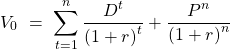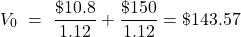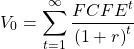Concept 76: Dividend Discount and Free-Cash-Flow-to-Equity Models | IFT World
101 Concepts for the Level I Exam

# Concept 76: Dividend Discount and Free-Cash-Flow-to-Equity ModelsDividend discount model: Value is estimated as the present value of expected future dividends plus the present value of a terminal value.A stock paid a $10 dividend last year. The next year’s dividend will be 8% higher and the stock will sell at$150 at year-end. Calculate the value of this stock if the required rate of return is 12%.

Solution:

D1 = D0 x (1 + dividend growth rate) = $10 x 1.08 =$10.8Free cash flow to equity model: Value is estimated as the present value of expected future free cash flow to equity. FCFE is the cash available to the firm’s equity holders after a firm meets all its other obligations.

FCFE = CFO – FCInv + Net borrowingEstimating the required rate of return for equity: CAPM is often used to calculate the required rate of return for a security.

Required rate of return = risk free rate + β [market risk premium]

Zoom Classes for Nov-23 Exam Starting Now!
This is default text for notification bar